This is a companion notebook for my blog post.

## Introduction

It is a known (among compiler developers) fact that checksums stored in the headers of Windows portable executable (PE) files are not actually checked by the operating system, with the exception of critical processes and libraries loaded into their address spaces (drivers, in particular). For this reason, it is not uncommon to come across a PE binary with an invalid checksum. In this notebook, a distribution of checksums collected from a typical Windows system is examined and compared to that for the checksums found in a mix of malware and benign modules from a known dataset.

Checksum is a hash function of the PE file contents (be it a rather imperfect one) and thus we can talk about collisions. The dataset contains collision counts computed for valid and invalid checksums separately.

Let us begin with the correct checksums

``````gdf <- read.csv("good.csv", header = FALSE, sep = ' ')
names(gdf) <- c("CheckSum", "Collision_Count")
summary(gdf)``````
``````##     CheckSum         Collision_Count
##  Min.   :     2611   Min.   :  1.00
##  1st Qu.:    68700   1st Qu.:  1.00
##  Median :   130188   Median :  1.00
##  Mean   :   750780   Mean   :  1.65
##  3rd Qu.:   371114   3rd Qu.:  2.00
##  Max.   :377287567   Max.   :118.00``````

and then proceed to the incorrect ones.

``````bdf <- read.csv("bad.csv", header = FALSE, sep = ' ')
names(bdf) <- c("CheckSum", "Collision_Count")
summary(bdf)``````
``````##     CheckSum         Collision_Count
##  Min.   :        0   Min.   :   1.00
##  1st Qu.:    68248   1st Qu.:   1.00
##  Median :   162137   Median :   1.00
##  Mean   :  4787647   Mean   :  24.98
##  3rd Qu.:   848965   3rd Qu.:   1.00
##  Max.   :377287567   Max.   :7135.00``````

As far as valid checksums go, the maximum (encountered) number of identical values is 118 and at least 50% of chechsums are unique, which hints at non-uniformity of distribution (possibly, a narrow peak coupled with long thin tails). With invalid checksums, the situation is a bit different: as many as 75% of values are non-repeating, but there is one with the whopping 7135 numbers of collisions. It tell us that this value plays a special role.

Utterly remarkable is the fact that the maximum value is the same for both valid and invalid checksums: `377287567` (`0x167CF38F`) despite the theoretical limit being `0xFFFFFFFF` (PE’s CheckSum field is 32-bit-wide). Normally, it would suggest a peculiarity in the checksum-computing algorithm imposing an artificial boundary; in this case, however, it is only a coincidence.

Below are high-level stats.

``````totg <- sum(gdf\$Collision_Count)
totb <- sum(bdf\$Collision_Count)
tot <- totg + totb
zerocs <- bdf[bdf\$CheckSum == 0, ]\$Collision_Count
cat(paste("Out of", tot, "binaries, there are\n"))
cat(paste0("   * ", totg, " with valid checksums (", round((totg/tot) * 100, 2), "%) and\n"))
cat(paste0("   * ", totb, " with malformed checksums (", round((totb/tot) * 100, 2), "%).\n"))
cat(paste0("Of the latter, ", zerocs, "(", round((zerocs/totb) * 100, 2), "%) have zero checksum."))``````
``````## Out of 64121 binaries, there are
##    * 56652 with valid checksums (88.35%) and
##    * 7469 with malformed checksums (11.65%).
## Of the latter, 7135(95.53%) have zero checksum.``````

Unsurprisingly, zero has turned out to be the “special” checksum value mentioned earlier. We will exclude zero checksums from consideration when constructing boxplots, histograms, and KDE plots as this value seems to have a distinctive meaning: the CheckSum field is intentionally left “blank” rather than being miscalculated.

Further computations require collision counts for correct and incorrect checksums combined in a single dataframe with an additional column indicating checksum validity.

``````gdf_v <- cbind(gdf, rep(1, dim(gdf)))
names(gdf_v) <- c(names(gdf), "Valid")

bdf_v <- cbind(bdf, rep(0, dim(bdf)))
names(bdf_v) <- c(names(bdf), "Valid")

df <- rbind(gdf_v, bdf_v)
df <- df[df\$CheckSum > 0, ]``````

In the interest of avoiding having to make humdrum inventions (e.g. wheels, functions that draw boxplots based on counts) let us present the dataframe in a more traditional form.

``````#copies every value df\$col_to_expand[i] (i = 1 to dim(df) ) counts_col[i] times
expand_column <- function(df, col_to_expand, counts_col) {
lst <- mapply(rep, df[col_to_expand], df[counts_col])
lst <- unlist(lst, recursive = FALSE)
lst
}

#turns integers into factors (generates proper plot labels automatically)
factorize_valid <- function(v) {
factor(v, levels = c(0, 1), labels = c("no", "yes"))
}

lstcs <- expand_column(df, "CheckSum", "Collision_Count")
lstvl <- expand_column(df, "Valid", "Collision_Count")

dfe <- data.frame(cbind(lstcs, lstvl))
names(dfe) <- c("CheckSum", "Valid")
dfe\$Valid <- factorize_valid(dfe\$Valid)``````

## Beware of Log Scale!!!

Take a look at the unscaled histogram plots.

``````h1<- ggplot(gdf, aes(x = CheckSum)) +
geom_histogram(bins = 50, fill = color_good, col = color_hist_border)
h2<- ggplot(bdf[bdf\$CheckSum > 0, ], aes(x = CheckSum)) +
geom_histogram(bins = 50, fill = color_bad, col = color_hist_border)
h1 + h2 + plot_annotation(title = "Completely Unscaled Checksum Histograms")``````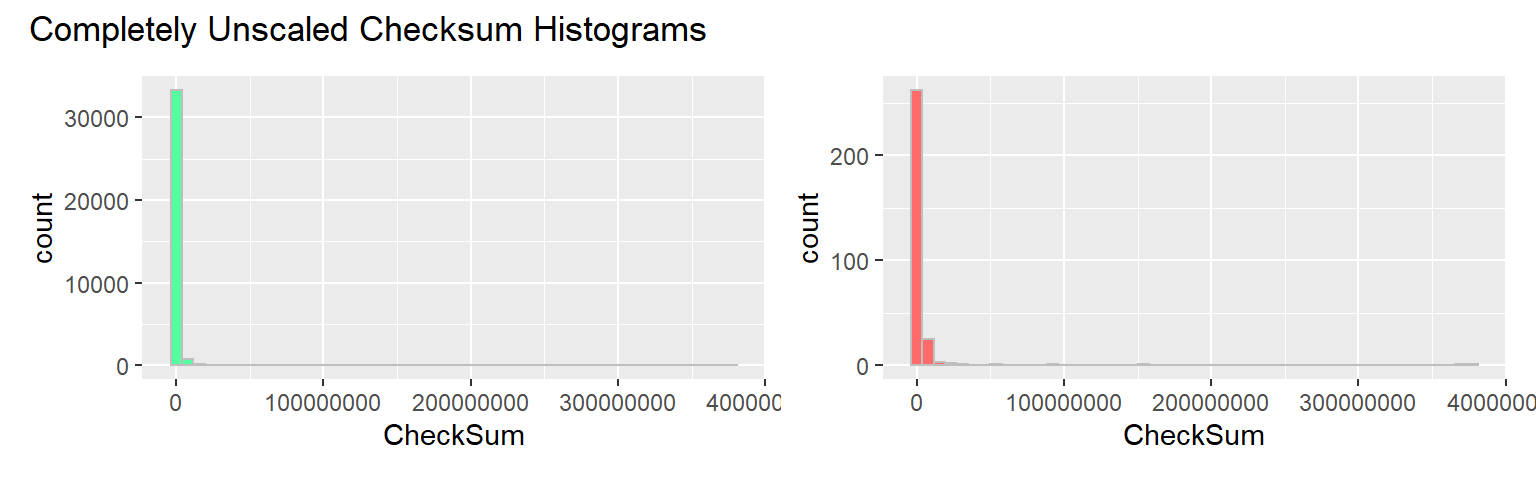The `CheckSum` value range is quite large with most of the weight concentrated towards the smaller numbers. Concerns of similar nature pertain to the frequencies, where a few large values dominate the range. This is why here and there we log-scale the axes: sometimes x, sometimes y, other times both. Naturally, it has side-effects; when `CheckSum` is log-scaled, for example, the right distribution tail will seem shorter than it actually is.

## Comparing Valid and Invalid Checksum Distributions

Let us now compare the distributions for valid and invalid checksums, starting with a boxplot as a tool.

``````mean1 <- mean(dfe[dfe\$Valid == factorize_valid(1), "CheckSum"])
mean0 <- mean(dfe[dfe\$Valid == factorize_valid(0), "CheckSum"])

ggplot(dfe, aes(x = CheckSum, y = Valid, group = Valid, fill = Valid)) +
geom_boxplot(outlier.size = 1) + geom_whisker_bar() +
#do not use stat_summary() as it seems to compute mean(log(x));
#meani = 10^mean(log10(dfe[dfe\$Valid == i, "CheckSum"])) will produce the same result
#stat_summary(fun = "mean", color = color_basic, fill = color_highlight, shape = bp_mean_shape) +
geom_point(mapping = aes(x = mean0, y = factorize_valid(0)),
color = color_highlight, shape = bp_mean_shape) +
geom_point(mapping = aes(x = mean1, y = factorize_valid(1)),
color = color_highlight, shape = bp_mean_shape) +
scale_x_log10() +
ggtitle("Boxplot for Valid and Invalid Checksums", subtitle = "(CheckSum Is in Log Scale)") +
xlab("Checksum") + ylab("Validity of Checksum")``````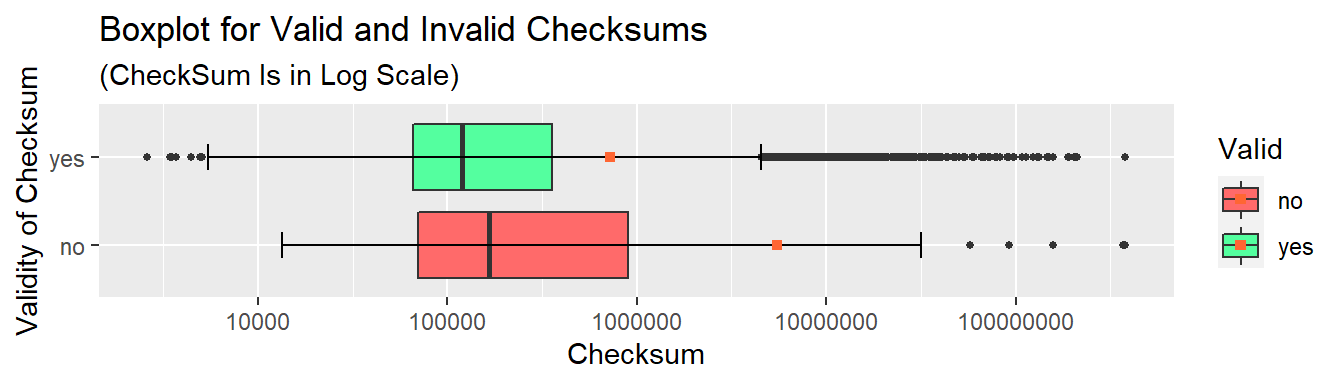Both distributions are heavily skewed, with long right tails, but the invalid checksum distribution is noticeably more spread-out (as evidenced by a wider interquartile range) and shifted towards higher values (take a note of whiskers’ positions). Overall, checksum values tend to reside “on the smaller side”, the fact easily explained by the way they are computed. As a result, the distribution is far from uniform, which renders the checksum a less than perfect candidate for a hash of the PE file's contents.

``````ggplot(dfe, aes(x = CheckSum, fill = Valid, col = Valid)) +
geom_density(lwd = 0.7, alpha = 0.5) +
scale_x_log10() +
ggtitle("Estimated PDFs for Valid and Invalid Checksums", subtitle = "(CheckSum Is in Log Scale)") +
xlab("Checksum") + ylab("Density") +
theme(legend.position = c(0.9, 0.8))``````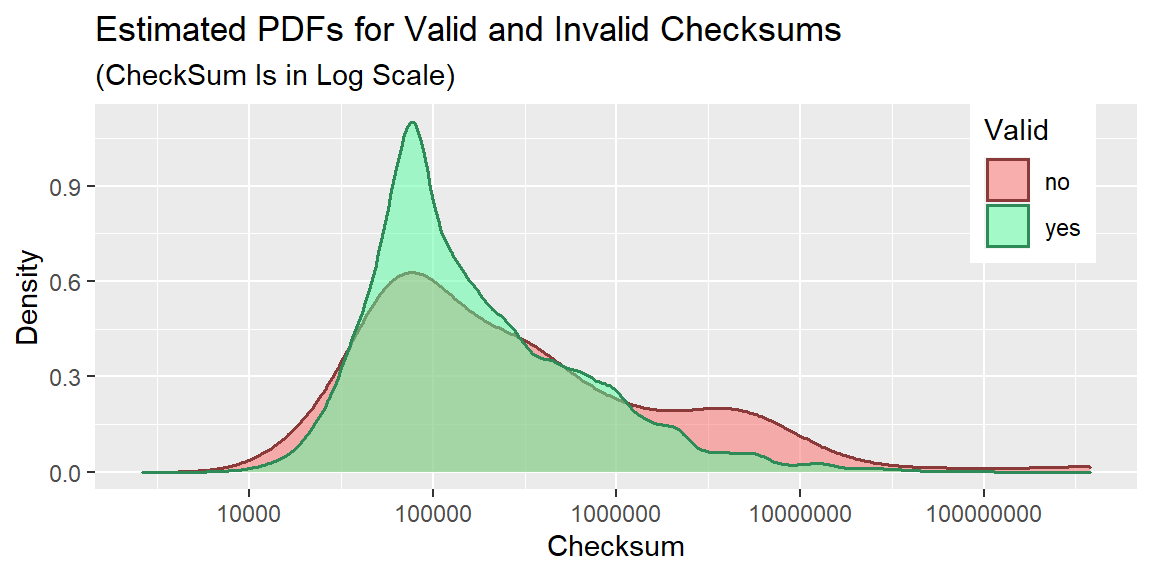Next, we present more detailed plots depicting the value distributions, but for valid and invalid checksums separately.

## Valid Checksums

### Collision Counts and Histogram for Checksum (Unscaled)

These plots show how skewed the distribution actually is.

``````egdf <- data.frame(expand_column(gdf, "CheckSum", "Collision_Count"))
names(egdf) <- c("CheckSum")

plot_collisions_and_historgam("Valid", gdf, egdf, color_good, color_good_highlight)``````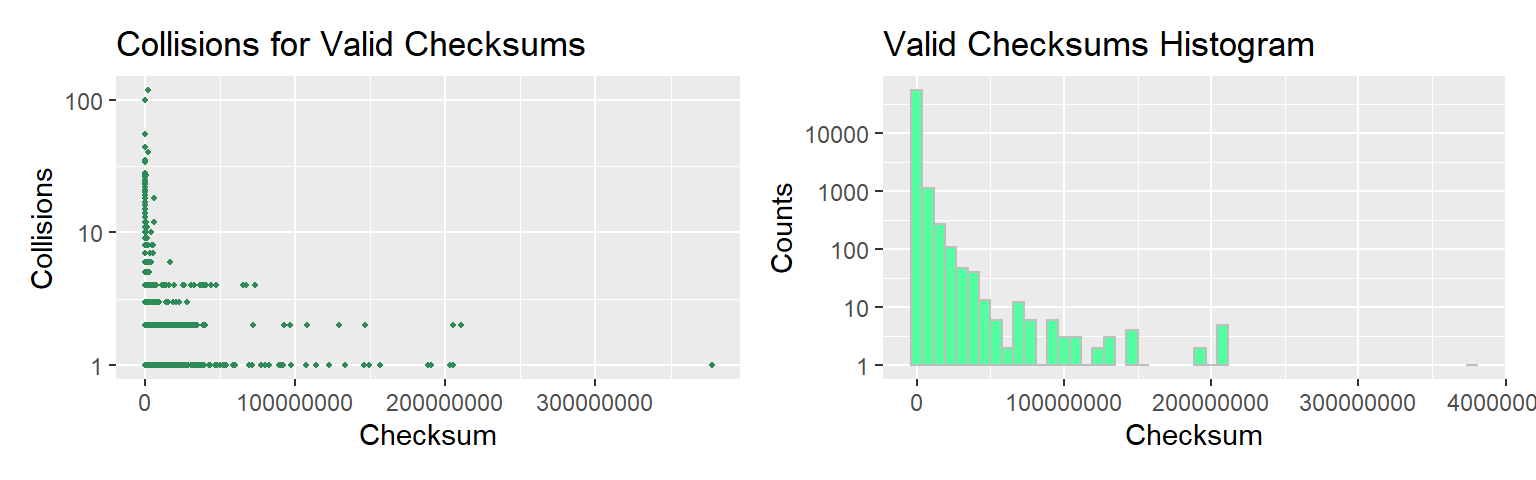### Collision Counts and Estimated PDF

``````plot_pdf_collisions_combined(gdf, egdf,
"Collisions and PDF for Valid Checksums",
color_good, color_good_highlight)``````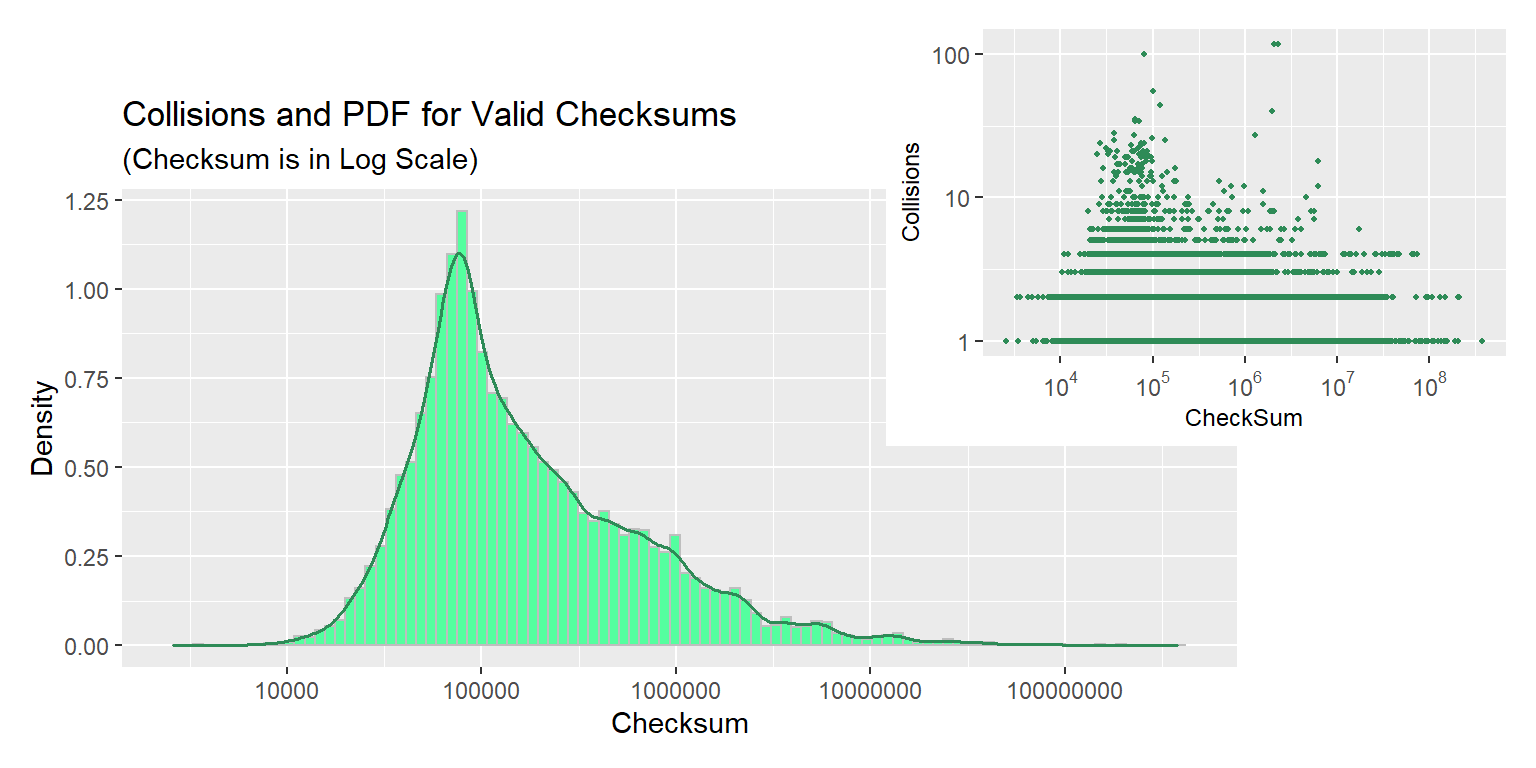Looking at the collision counts plot, one would expect a bimodal PDF; however, the density plot assumes a different shape on account of the second peak being outweighed by lower values “from the same bin”. The relatively narrow peak we predicted earlier is clearly visible, while the long thin tail is less so on account of its length having been concealed by logarithmic scale. Such a PDF shape implies that the probabiity of a random checksum value falling in the tight region around 100000 is significantly higher as compared to other regions in the range.

## Invalid Checksums

### Collision Counts and Histogram for Checksum (Unscaled)

``````ebdf <- data.frame(expand_column(bdf[bdf\$CheckSum > 0, ], "CheckSum", "Collision_Count"))
names(ebdf) <- c("CheckSum")

plot_collisions_and_historgam("Invalid", bdf[bdf\$CheckSum > 0, ], ebdf,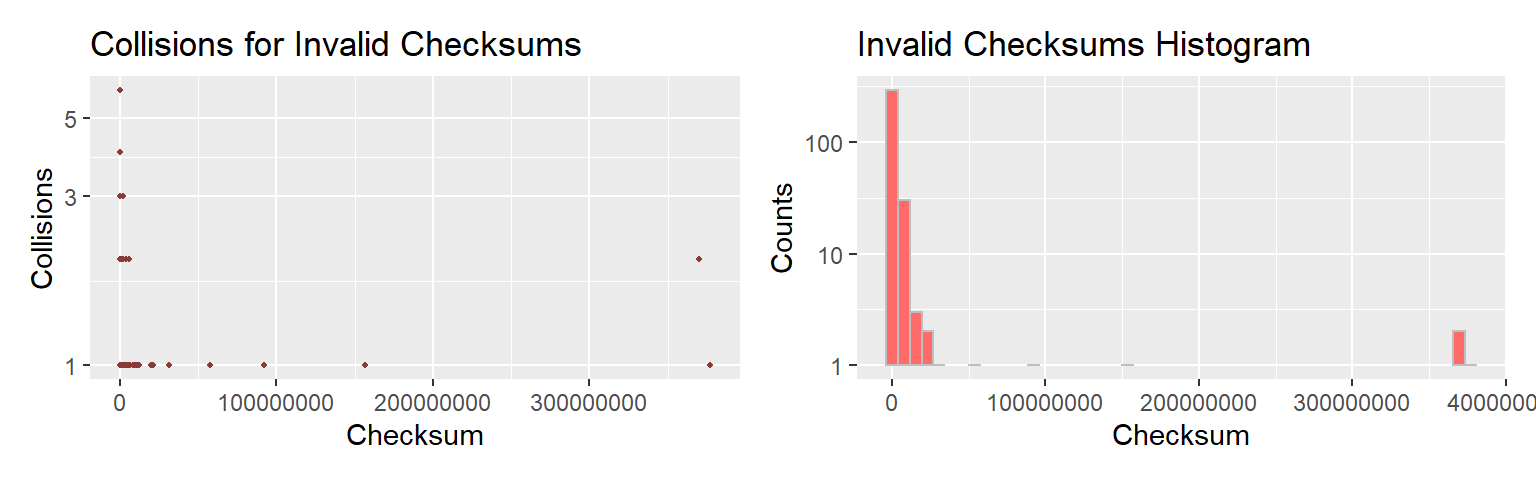### Collision Counts and Estimated PDF

``````plot_pdf_collisions_combined(bdf[bdf\$CheckSum > 0, ], ebdf,
"Collisions and PDF for Invalid Checksums",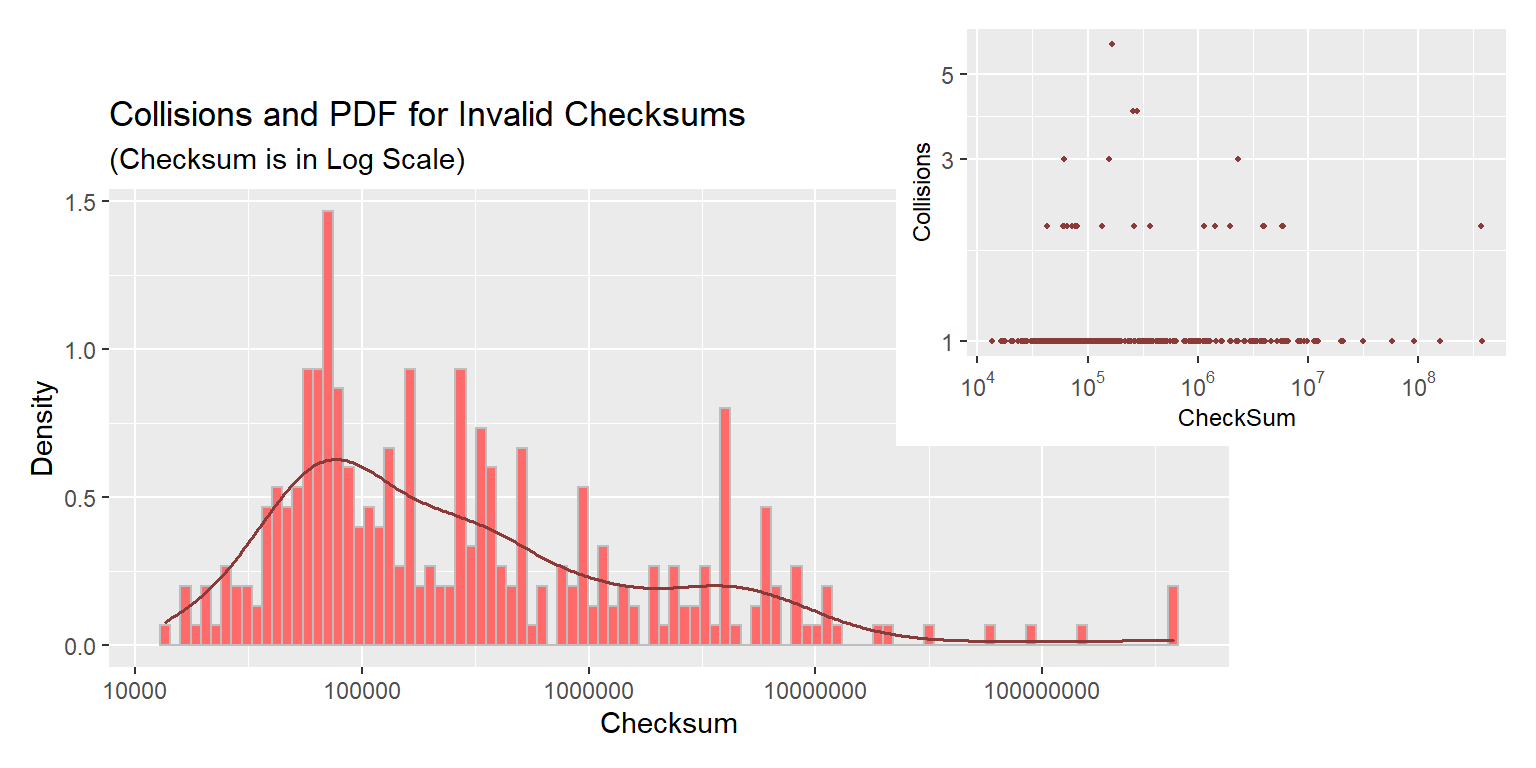At first glance, collision counts plot looks very similar to that for valid checksums, but the histogram and KDE show a different picture. The estimated PDF is much flatter giving a decent probability of occuring to a wider range of values. Keep in mind, however, that there are significantly fewer binaries with incorrect non-zero checksum in the dataset, which makes one wonder if the sample in question can be deemed representative and what the plot would look like were we to obtain more data.

``````cat(paste("Number of binaries with non-zero invalid checksum:", paste0(dim(ebdf), ".\n")))
cat(paste("Number of binaries with valid checksum:", paste0(dim(egdf), ".\n")))``````
``````## Number of binaries with non-zero invalid checksum: 334.
## Number of binaries with valid checksum: 56652.``````

## Valid and Invalid CheckSums, Combined

For the reason specified above, the combined checksum is dominated by its valid constituent and, therefore, the plots should bring no surprises.

In order to obtain a dataframe containing both valid and invalid checksums, we perform an outer join on the `CheckSum` column with the subsequent summation of collision counts.

``````dfm <- merge(gdf[, c("CheckSum", "Collision_Count")],
bdf[bdf\$CheckSum > 0, c("CheckSum", "Collision_Count")],
by = "CheckSum", all = TRUE)

dfm\$Collision_Count_Good[is.na(dfm\$Collision_Count_Good)] <- 0

dfme <- data.frame(expand_column(dfm, "CheckSum", "Collision_Count"))
names(dfme) <- c("CheckSum")``````

### Boxplot for Combined Checksums

``````ggplot(dfme, aes(x = CheckSum)) +
geom_boxplot(fill = color_basic) + geom_whisker_bar() +
geom_point(mapping = aes(x = mean(CheckSum), y = 0), color = color_highlight,
shape = bp_mean_shape, size = 2) +
scale_x_log10() +
ggtitle("Checksums Boxplot", subtitle = "(CheckSum Is in Log Scale)") +
xlab("Checksum") + ylab("")``````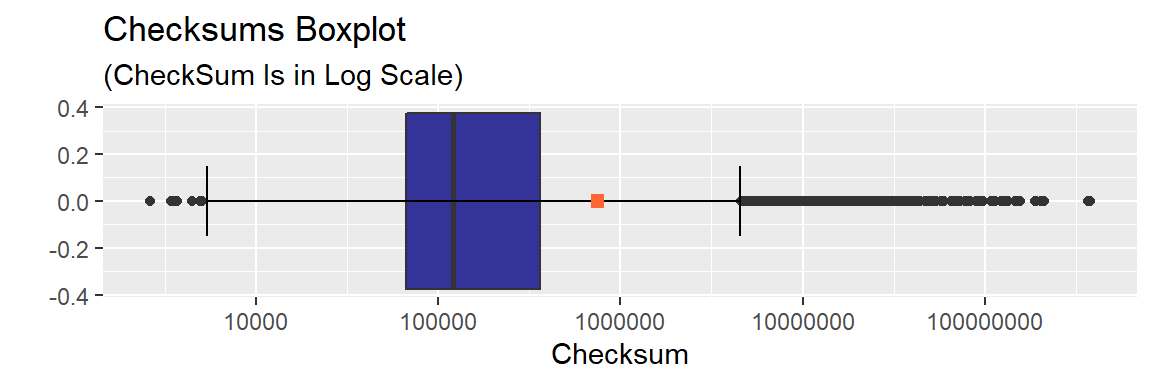### Collision Counts and Histogram for Combined Checksum (Unscaled)

``````ebdf <- data.frame(expand_column(bdf[bdf\$CheckSum > 0, ], "CheckSum", "Collision_Count"))
names(ebdf) <- c("CheckSum")

plot_collisions_and_historgam("All", dfm, dfme,
color_basic, color_highlight)``````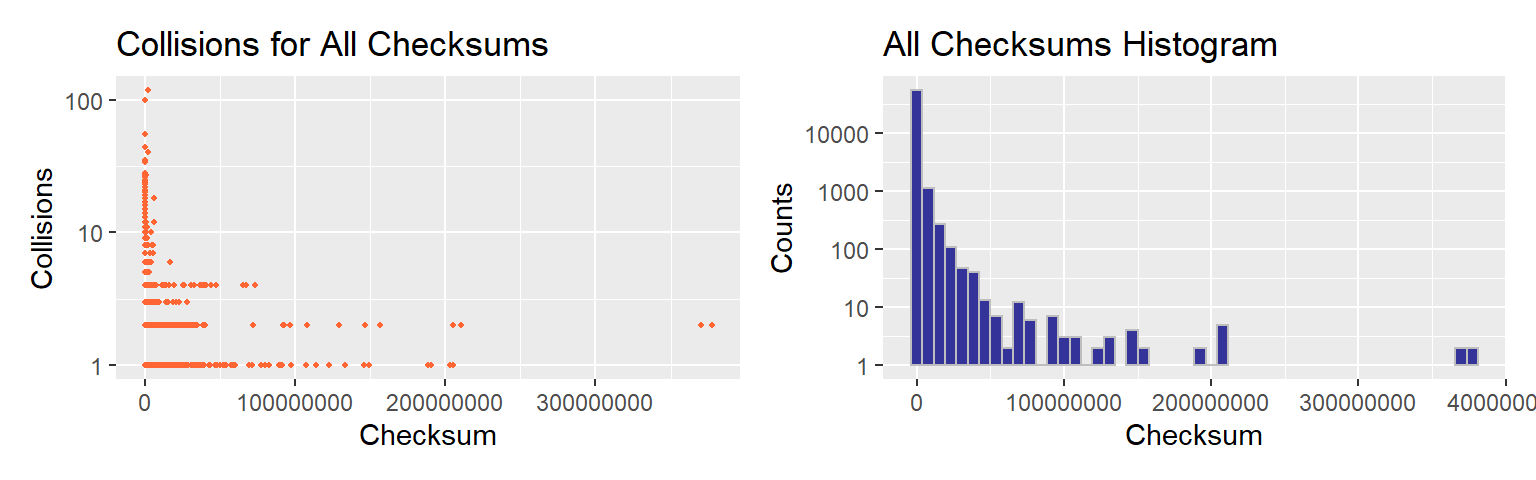### Histogram and Estimated PDF for Combined Checksum

``````plot_pdf_collisions_combined(dfm, dfme,
"Collisions and PDF for All Checksums",
color_basic, color_highlight)``````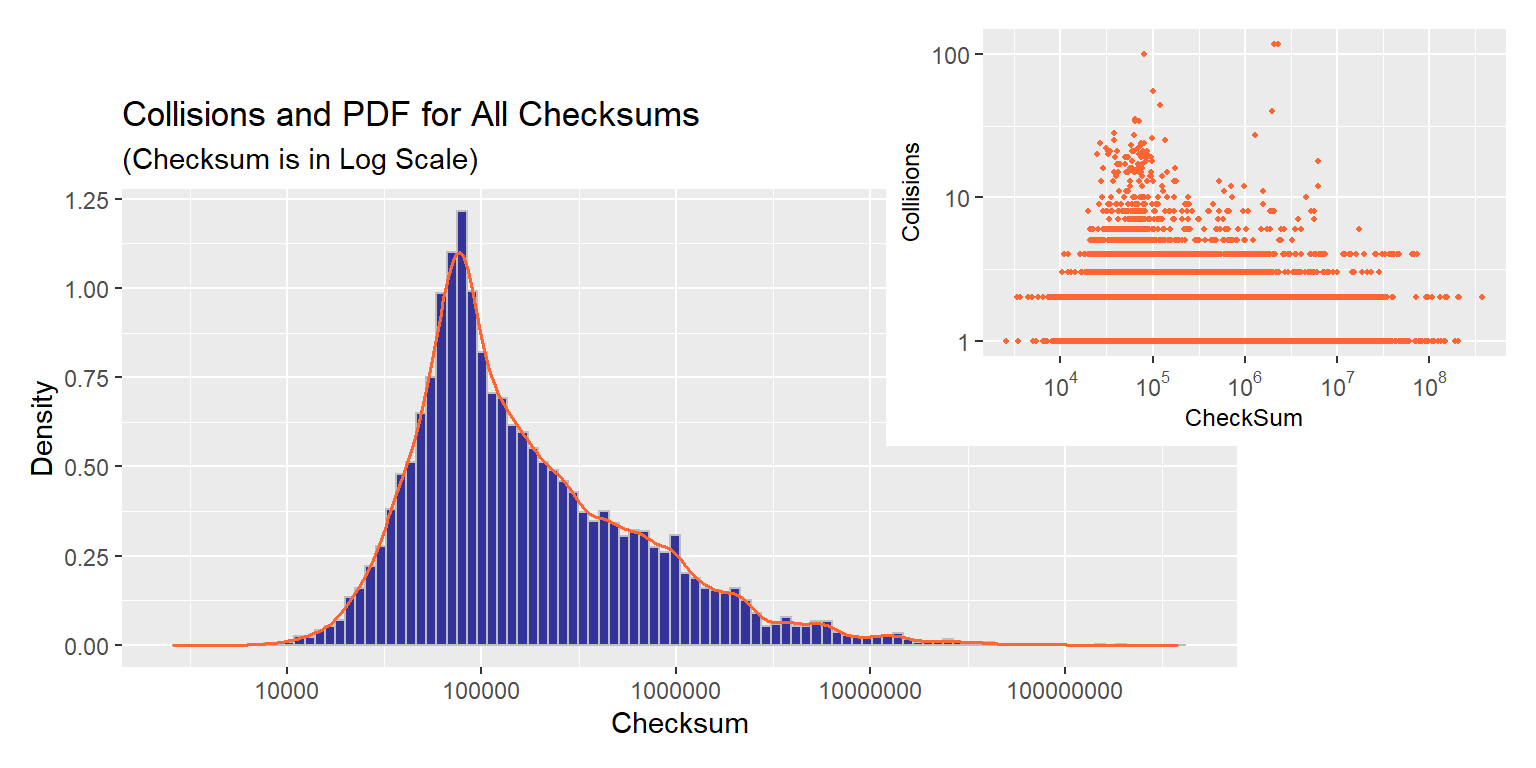## Benign & Malicious PE Files Dataset

We will use Mauricio Jara’s Benign & Malicious PE Files dataset, a collection of values (and a few derivatives thereof useful for malware detection) extracted from headers of PE files. The data was obtained by parsing binaries found in two Windows installations and an assortment of malware requested from VirusShare. Among the PE fields is `CheckSum` and this is the only field we are going to use. That is, for every binary, we are given a `CheckSum` value (as stored in its PE header) and whether this module is malicious or benign (clean). Unfortunately, it is unknown if the given checkum is valid.

On a brighter note, unlike in the previous dataset, even if we consider positive checksums only, there are enough datapoints of both kinds to avoid bias towards an over-represented class (in the context of our problem, at least).

``````mal_df <- read.csv("dataset_malwares.csv", header = TRUE, sep = ',')
print(paste("Number of clean binaries with non-zero checksum",
dim(mal_df[mal_df\$CheckSum > 0 & mal_df\$Malware == 0, ])))
print(paste("Number of malware binaries with non-zero checksum",
dim(mal_df[mal_df\$CheckSum > 0 & mal_df\$Malware == 1, ])))``````
``````##  "Number of clean binaries with non-zero checksum 4889"
##  "Number of malware binaries with non-zero checksum 9828"``````

First and foremost, let us check if the maximum value of `CheckSum` is the same as in the dataset considered previously.

``summary(mal_df\$CheckSum)``
``````##       Min.    1st Qu.     Median       Mean    3rd Qu.       Max.
##          0       4476     205533  115491079     701512 4294967295``````

Alas! It is not. As it has already been mentioned, it is by coincidence that `0x167CF38F` happens to be the maximum value of both, valid and invalid, checksum sets.

Let us take a look at the distribution of checksums in this dataset.

``````plot_hist_pdf(mal_df[mal_df\$CheckSum > 0, ],
"Distribution of Checksums in Benign & Malicious PE Files Dataset",
color_basic, color_highlight)``````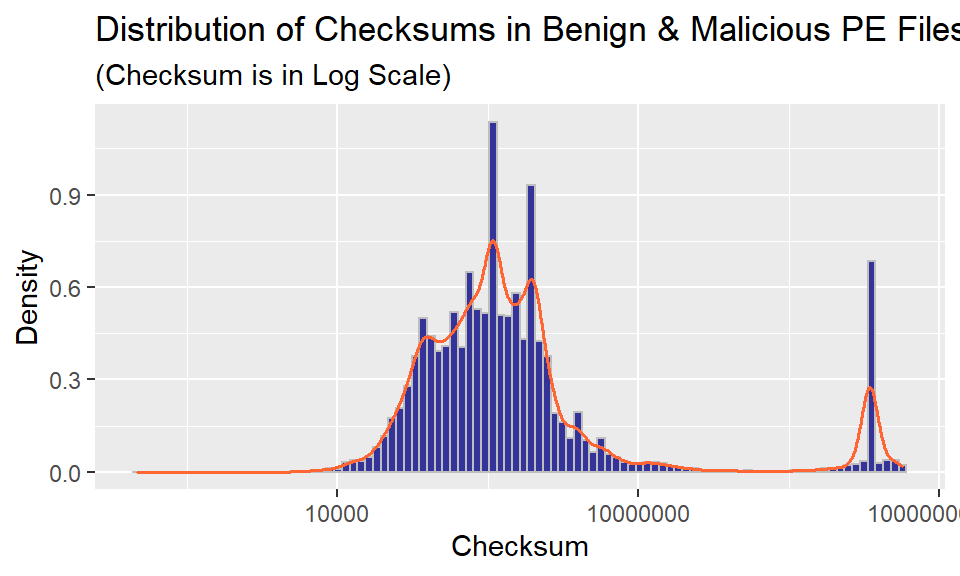The plot is unlike anything we have seen up to this point (in this notebook). Why not dissect the distribution for the purpose of understanding it better? The way to go about it is to examine clean and malicious binaries separately.

### Distribution of Clean Binaries’ Checksums

``````plot_hist_pdf(mal_df[mal_df\$CheckSum > 0 & mal_df\$Malware == 0, ],
"Distribution of Checksums for Clean Binaries",
color_good, color_good_highlight,
"Validity of Checksums is Unknown")``````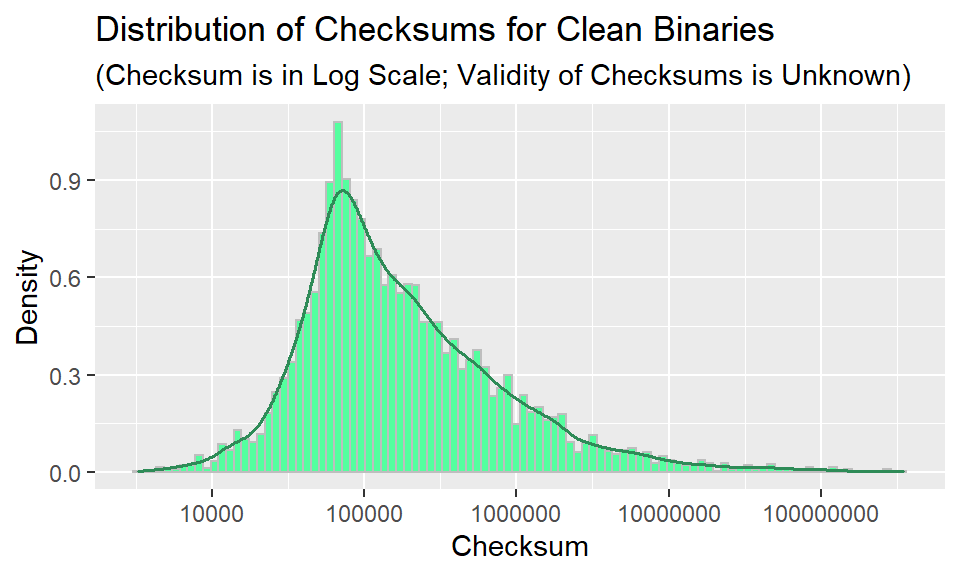Either all green plots look alike or there is a definite similarity between distributions of correct checksums and checksums stored in the PE headers of clean binaries.

### Comparing CheckSum Distributions: Valid Checksums and Checksums of Benign Binaries

Putting apples and oranges in one basket, we combine binaries with correct checksum from the first dataset and clean software from the second in a single dataframe.

``````#Malware and Benign PE Dataset
benign_df <- mal_df[mal_df\$CheckSum > 0 & mal_df\$Malware == 0, c("CheckSum", "Malware")]
names(benign_df) <- c("CheckSum", "Type") #rename Malware (always 0) to Type
#our Collision Counts for Valid and Invalid Checksums Dataset
egdf_t <- cbind(egdf, rep(1, length(egdf))) #assign all the datapoints Type 1
names(egdf_t) <- c("CheckSum", "Type")
apor_df <- rbind(benign_df, egdf_t)

factorize_type <- function(type) {
factor(type, levels = c(0, 1), labels = c("Clean", "Valid Checksum"))
}

apor_df\$Type <- factorize_type(apor_df\$Type)

#plotting
mean_clean <- mean(apor_df[apor_df\$Type == factorize_type(0), "CheckSum"])
mean_valid <- mean(apor_df[apor_df\$Type == factorize_type(1), "CheckSum"])

ggplot(apor_df, aes(x = CheckSum, y = Type, group = Type)) +
geom_violin(width = 1, fill = color_good) + geom_whisker_bar() +
geom_boxplot(width = .2, fill = color_basic, outlier.shape = NA) +
geom_point(mapping = aes(x = mean_clean, y = factorize_type(0)),
color = color_highlight, shape = bp_mean_shape) +
geom_point(mapping = aes(x = mean_valid, y = factorize_type(1)),
color = color_highlight, shape = bp_mean_shape) +
scale_x_log10() +
ggtitle("Binaries with Valid Checksums vs Clean Binaries", subtitle = "(CheckSum Is in Log Scale)") +
xlab("Checksum") + ylab("Validity of Checksum")``````